### Blog Options

#### Archive

<< September 2019 >>

# Blog

Most Recent Post  |  Index List

#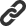Drawing math formulae generated with TMS Analytics & Physics library

Bookmarks:

#### Monday, January 07, 2019

TMS Analytics & Physics developing library contains many algorithms for manipulating with symbolic math expressions. For example, it is able to evaluate symbolic derivatives of complicated expressions, approximate functions with symbolic, user-defined basis and so on. The result of any such manipulation is another symbolic expression. Many efforts in last versions has been spent for simplifying result expressions. The expression simplification required for presenting the result in shorted and readable form. For example, the derivative of ‘y^(x+1)’ by ‘x’ can be formally written as ‘(x+1)*y^(x+1-1)’ and this is correct answer, but after simplification it would be simpler for reading – ‘(x+1)*y^x’.

Nevertheless, all symbolic algorithms manipulate with math expressions, presented as plain Unicode strings. The main reason for this is providing simple interface for using math formulae inside Delphi programs. Presenting math expressions as plain strings requires using special syntax for different math structures. For example, power expression written with power operator ‘x^y’, vector expression written with brackets ‘[x y z]’ and so on. Such syntax is not ‘natural’ for math formula notation and so, result expressions are not suitable for ‘human reading’.

New version 2.7 of TMS Analytics and Physics pack contains new tools to convert symbolic expressions, generated with the library, to TeX format (https://en.wikibooks.org/wiki/LaTeX/Mathematics). This format is widely used for creating scientific articles and documents. There are many free tools for drawing math formula, presented in TeX format in desktop and Web applications, converting documents to PDF or DOC format and so on.

Let us consider as symbolic expressions, generated with the library, can be drawn with Web browser as math formulae. First, we need to convert the expression to the TeX format. Here is an example of code for converting expressions:

var
f, texf: string;
converter: TAnalyticsConverter;
begin
f:= 'A*sin(n*x)+B/2*e^(m*y)';
converter:= TAnalyticsTeXConverter.Create;
try
texf:= converter.Convert(f);
except on E: Exception do
// Show exception message...
end;

// Using formula in TeX format...
end;

In the code above an instance of the TAnalyticsTeXConverter class created. This class has the Convert method for converting symbolic expressions to the TeX format. For the expression, result string in TeX format is the following:

{{{A}cdot{{sin}left({{n}cdot{x}}right)}}+{{frac{B}{2}}cdot{{e}^{{m}cdot{y}}}}}

Expression in TeX format can be then drawn in Web browser using, for example, MathJax CDN service (https://en.wikipedia.org/wiki/MathJax). For doing this we can generate a file with the following HTML code:
<!DOCTYPE html>
<html>
<script type="text/javascript" src="http://cdn.mathjax.org/mathjax/latest/MathJax.js?config=TeX-AMS-MML_HTMLorMML"></script>
<title> TMS Analytics & Physics 2.7 </title>
<body>
$$XXX$$
</body>
</html>


where XXX must be replaced with the TeX formula. Launching browser for the generated HTML file the formula will be drawn. For the formula above, we get: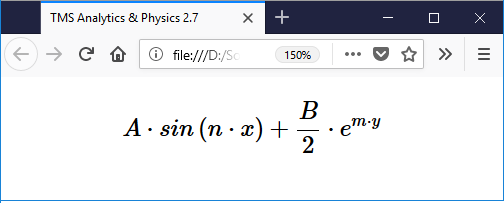The converter to TeX format supports all formulae, satisfying the syntax of the library. All types of expressions can be converted and rendered, including implicit derivative operators, special functions and operators (sum, square root and so on).

There are some other examples of complicated formula, rendered in Web browser with the described approach.

- Implicit derivative expression: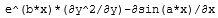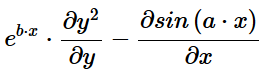- Expression with indexed data:
A[n-1]*sin(n*Pi*x)+B[m+1]*e^(m*y)+1/2*M[i][j]/(i+j)!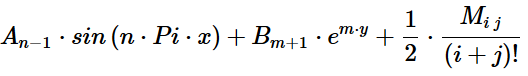- Matrix/vector expression: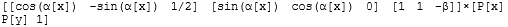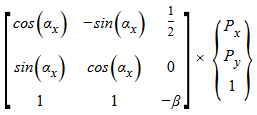- Special function/operator expression: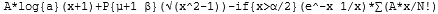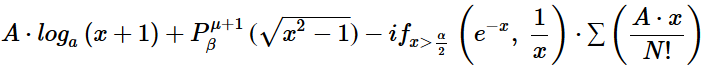As can be seen from the pictures above, all rendered formulae presented in natural math format and are easy to read and understand. Thus, all symbolic results, evaluated with the library, can be shown to the user as math formulae.

The version 2.7 is already available. Source code of the example application can be downloaded from here.

Bruno Fierens

Bookmarks: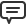#### This blog post has received 6 comments.1. Monday, January 07, 2019 at 5:08:46 PM

This is awesome!

Price Rhett2. Tuesday, January 08, 2019 at 12:04:42 PM

Nice. Do you plan to provide direct drawing to TCanvas in the future ?

Riche John3. Tuesday, January 08, 2019 at 5:03:03 PM

There are no immediate plans to support direct drawing. The library is for symbolic manipulations, not for drawing formulae. The main goal of conversion to the TeX format is providing possibility of using other 3rd party libraries to render formulae got with the TMS Analytics

Bruno Fierens4. Friday, January 11, 2019 at 2:13:21 AM

Now looking for a TeX rendering component in FireMonkey!

van der Linden Scott5. Thursday, January 17, 2019 at 11:31:00 AM

Update: I''m not finding any TeX components for Delphi, VCL or FMX. Can anyone point me at one?

van der Linden Scott6. Sunday, January 20, 2019 at 7:01:54 PM

Another brilliant development from TMS Components. I wish I had all of this when I was computing annuity rates and life assurance rates for a life company in ...1976. Casio programmable handhelds came out so that ''automated'' valuations at the time.

Well done all.

Francis

FCII F G Moore

 Add a new comment: Author: Email: You will receive a confirmation mail with a link to validate your comment, so please use a valid email address. Comment: All fields are required.

Most Recent Post  |  Index List